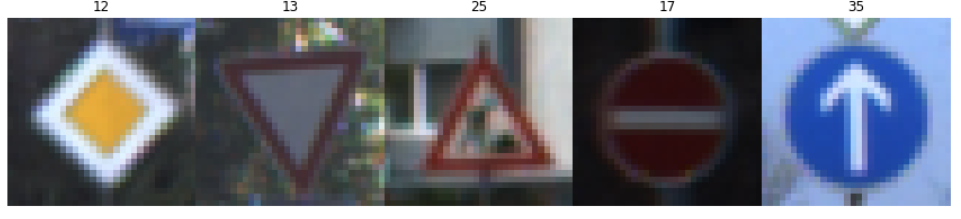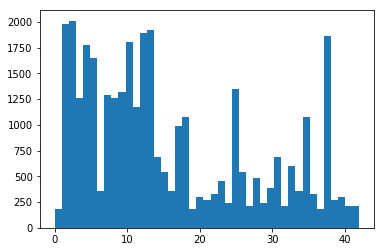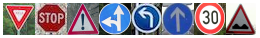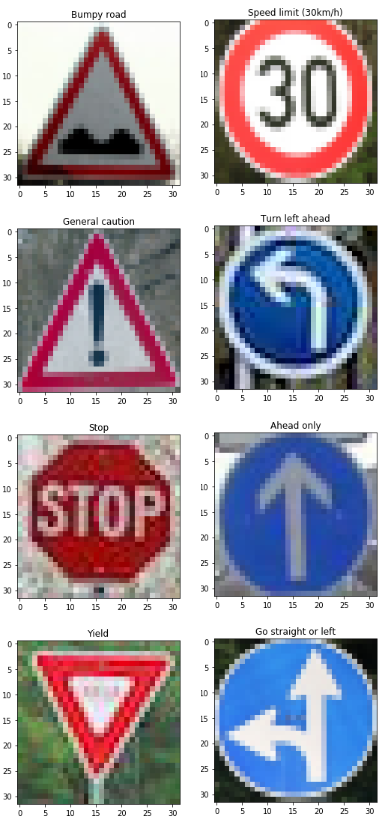# 1. Overview

In this project, I have implemented a classifier using deep neural networks, convolutional neural networks and transfer learning to classify traffic signs. I have trained a model so that it can decode traffic signs from natural images by using the German Traffic Sign Dataset. After the model is trained, I have tested my model program on new images of traffic signs collected from the web.

The steps of this project are the following:

• Load the data set (see below for links to the project data set)
• Explore, summarize and visualize the data set
• Design, train and test a model architecture
• Use the model to make predictions on new images
• Analyze the softmax probabilities of the new images
• Summarize the results with a written report

# 2. Data Set Summary & Exploration

This is a pickled dataset in which we’ve already resized the images to 32x32.

## Provide a basic summary of the data set

I used the numpy library to calculate summary statistics of the traffic signs data set:

• The size of training set is 34799
• The size of validation set is 4410
• The size of test set is 12630
• The shape of a traffic sign image is (32, 32, 3)
• The number of unique classes/labels in the data set is 43

## Include an exploratory visualization of the dataset

Here is an exploratory visualization of the data set. It pulls in a random set of 5 images.I also detail the dataset structure by plotting the occurrence of each image class to get an idea of how the data is distributed.# 3. Design and Test a Model Architecture

My final model consisted of the following layers:

Layer Description
Input 32x32x3 RGB image
Convolution 5x5 2x2 stride, valid padding, outputs 28x28x6
RELU
Max pooling 2x2 stride, outputs 14x14x6
Convolution 5x5 2x2 stride, valid padding, outputs 10x10x16
RELU
Max pooling 2x2 stride, outputs 5x5x6
Fully connected input 400, output 120
RELU
Dropout 50% keep
Fully connected input 120, output 84
RELU
Dropout 50% keep
Fully connected input 84, output 43

To train the model, I used an LeNet for the most part that was given, but I added a dropout function for deleteing the fully connected layer’s output. I used the AdamOptimizer with a learning rate of 0.001. The epochs used was 30 while the batch size was 64. Other important parameters I learned were important was the number and distribution of additional data generated.

My final model results were:

• Train Accuracy = 0.999
• Valid Accuracy = 0.968
• Test Accuracy = 0.946

# 4. Test a Model on New Images

Here are five German traffic signs that I found on the web:The model was able to correctly guess 8 of the 8 traffic signs, which gives an accuracy of 100%.For the first image, the model is relatively sure that this is a Bumpy road (probability of 0.996), and the image does contain a bumpy road. The top 3 soft max probabilities were:

Probability Prediction